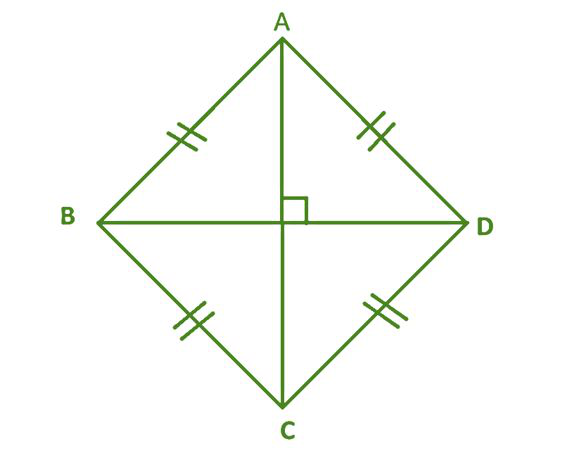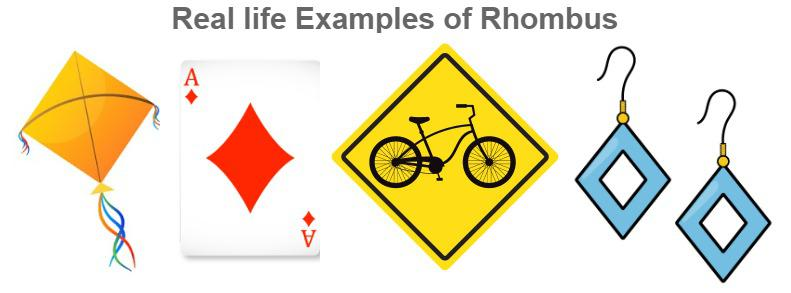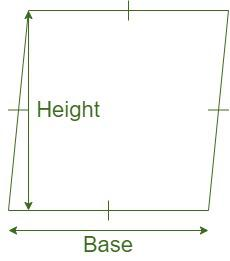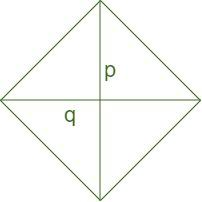Open in App
Not now

# Rhombus

• Last Updated : 09 Nov, 2022

A rhombus is a quadrilateral with all four sides equal and opposite sides parallel to each other, also its opposite angles are equal. Any rhombus can be considered a parallelogram, but not all parallelograms are considered rhombus. Similarly, all squares can be considered rhombuses, but not all rhombuses are considered squares. Diagonals of a rhombus are orthogonal bisectors of each other.

## What is a Rhombus?

Rhombus is a special case of parallelogram where the adjacent sides are equal in length and also the diagonals bisect each other at right angles. We can also state that a rhombus is actually a square only when all its angles are equal to 90 degrees. In the figure below, we can see that AB = BC = CD = DA. Also, the diagonals AC and BD bisect each other at a right angle.## How is a Rhombus Different from a Square?

Rhombus and Square both are Equilateral Quadrilaterals, i.e. both have equal all four sides. The difference between them is that all angles in a square are equal and right angles, but on the other hand all the angles of a rhombus need not be equal.

Hence, Rhombus with right angles is called a Square.

Thus, “Every Square is a Rhombus but all Rhombus are not Squares.”

## Real-Life Examples of Rhombus

Rhombus is a very common shape and can be seen in a variety of objects which we use in our daily lives. Various Rhombus-shaped objects are Jewelry, Kites, Sweets, Furniture, etc.## Properties of a Rhombus

Few of the properties of the Rhombus are given below:

• All the sides of a rhombus are equal. In fact, it is just a parallelogram with equal adjacent sides.
• All Rhombus has two diagonals, which connect the pairs of opposite vertices. A rhombus is symmetrical along both its diagonals. The diagonals of a rhombus are perpendicular bisectors to each other.
• In the event of all the angles of a rhombus being equal, it is called a square.
• The diagonals of a rhombus would always bisect each other at a 90 degrees angle.
• Not only do the diagonals bisect each other, but they also bisect the angles of a rhombus.
• The two diagonals of a rhombus divide it into four right-angled congruent triangles.
• There cannot be a circumscribing circle around a Rhombus.
• It is impossible to have an inscribing circle inside a rhombus.

## Rhombus Formulas

The two important formulas for Rhombuses are:

• Area of a rhombus, A = 1/2 × d1 × d2, where d1 and d2 are diagonals of a rhombus.
• The perimeter of a rhombus, P = 4 × a, where a is the side

## Area of a Rhombus

Area of Rhombus is the space enclosed by all four boundaries of Rhombus it is measured in unit squares. There are two ways of finding Areas of a Rhombus which are discussed below

### Area of Rhombus when Base and Altitude are given

When the Base and Altitude of a Rhombus are given then its area is calculated by the formula

Area = Base × Height### Area of Rhombus when both Diagonals are given

Area of a rhombus is equal to half the product of the lengths of both diagonals. Hence, if p and q denote the lengths of diagonals of a rhombus, its

Area = 1/2 × p × q.

where,

p is one diagonal

q is second diagonal## Perimeter of a Rhombus

Perimeter of a rhombus is defined as the sum of all its sides. Since all the sides of a rhombus are equal in length, it can be said that the Perimeter of a Rhombus is four times the length of one side. Thus, if s denotes the length of a side of a rhombus, its perimeter = 4s.

Perimeter = 4×s

where s is the side of Rhombus

## Solved Examples on Rhombus

Example 1: MNOP is a rhombus. If diagonal MO = 29 cm and diagonal NP = 14 cm, What is the area of rhombus MNOP?

Solution:

Area of a rhombus = (d1)(d2)/2

Substituting the lengths of diagonals in the above formula, we have:

A = (29)(14)/2 = 406/2 = 203 cm2

Area of rhombus MNOP = 203 cm2

Example 2: ABCD is a rhombus. The perimeter of ABCD is 40, and the height of rhombus is 12. What is the area of ABCD?

Solution:

Perimeter = 40 cm

Perimeter = 4 × side

40 = 4×side

side(base) = 10 cm

height = 12 cm (given)

Area = base × height

= 10×12 = 120 cm2

Thus, Area of rhombus ABCD is equal to 120 square cm

Example 3: Find the area of a rhombus with diagonal lengths of (2x+2) and (4x+4) units.

Solution:

We know, Area of a rhombus = (d1)(d2)/2

Substituting the lengths of diagonals in the above formula, we have:

A ==== (4x2 + 8x + 4) unit2

Example 4: Find the area of a rhombus if its diagonal lengths arecm andcm.

Solution:

We know, Area of a rhombus = (d1)(d2)/2

Substituting the lengths of diagonals in the above formula, we have:

A ==cm2

## FAQs on Rhombus

Question 1: Is Rhombus considered a regular polygon?

No, Rhombus is not considered a regular polygon, because a regular polygon have all its angles equal but Rhombus does not have all its angle equal.

Question 2: Is Every Square can be called Rhombus?

Yes, every Square can be considered as Rhombus. Square are considered as special case of a Rhombus If all the angles of a Rhombus are right angles, then it is considered as a Square.

Question 3: Write the Difference between a Parallelogram and a Rhombus.

The main difference between a parallelogram and a rhombus is that in a Rhombus all four sides are equal whereas a Parallelogram has only opposite sides equal in length.

Question 4: What is the Sum of all the Interior Angles in a Rhombus?

A Rhombus is a Quadrilateral and it has four angles. The sum of all the interior angles in a Rhombus is 360°.

Question 5: Are All Angles in a Rhombus 90°?